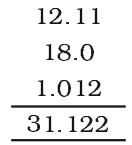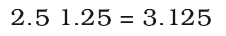# Significant Figures

The reliability of a measurement is indicated by the number of digits used to represent it. To express it more accurately we express it with digits that are known with certainty. These are called significant figures. They contain all the certain digits plus one doubtful digit in a number.

## Rules for Determining the number of significant Figures:

• All non-zero digits are significant.
For example, at 285 cm, there are three significant figures and in 0.25 mL, there are two significant figures.
• Zeros preceding to first non-zero digit are not significant. Such zero indicates the position of the decimal point.
Thus, 0.03 has one significant figure and 0.0052 has two significant figures.
• Zeros between two non-zero digits are significant. Thus, 2.005 has four significant figures.
• Zeros at the end or right of a number are significant provided they are on the right side of the decimal point.
For example, 0.200 g has three significant figures.
• But, if otherwise, the terminal zeros are not significant if there is no decimal point.
For example, 100 has only one significant figure, but 100. has three significant figures and 100.0 has four significant figures.
• Addition and Subtraction of Significant Figures: The result cannot have more digits to the right of the decimal point than either of the original numbers.Here, 18.0 has only one digit after the decimal point and the result should be reported only up to one digit after the decimal point which is 31.1.

• Multiplication and division of Significant Figures: In these operations, the result must be reported with no more significant figures as are there in the measurement with the few significant figures.Since 2.5 has two significant figures, the result should not have more than two significant figures, thus, it is 3.1.